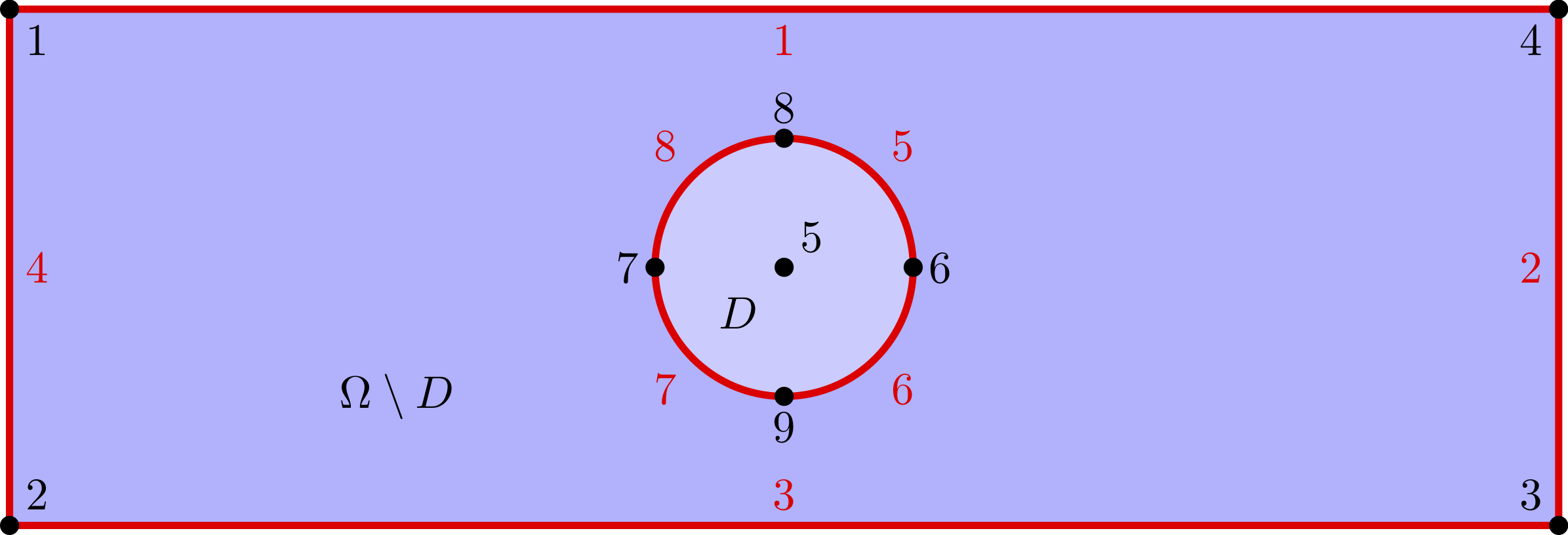# Creating Firedrake-compatible meshes in Gmsh¶

The purpose of this demo is to summarize the key structure of a gmsh.geo file that creates a Firedrake-compatible mesh. For more details about Gmsh, please refer to the Gmsh documentation. The Gmsh syntax used in this document is for Gmsh version 4.4.1 .

As example, we will construct and mesh the following geometry: a rectangle with a disc in the middle. In the picture, numbers in black refer to Gmsh point tags, whereas numbers in read refer to Gmsh curve tags (see below).The first thing we define are four corners of a rectangle. We specify the x,y, and z(=0) coordinates, as well as the target element size at these corners (which we set to 0.5).

Point(1) = {-6,  2, 0, 0.5};
Point(2) = {-6, -2, 0, 0.5};
Point(3) = { 6, -2, 0, 0.5};
Point(4) = { 6,  2, 0, 0.5};


Then, we define 5 points to describe a circle.

Point(5) = { 0,  0, 0, 0.1};
Point(6) = { 1,  0, 0, 0.1};
Point(7) = {-1,  0, 0, 0.1};
Point(8) = { 0,  1, 0, 0.1};
Point(9) = { 0, -1, 0, 0.1};


Then, we create 8 edges: 4 for the rectangle and 4 for the circle. Note that the Gmsh command Circle requires the arc to be strictly smaller than $$\pi$$.

Line(1) = {1, 4};
Line(2) = {4, 3};
Line(3) = {3, 2};
Line(4) = {2, 1};
Circle(5) = {8, 5, 6};
Circle(6) = {6, 5, 9};
Circle(7) = {9, 5, 7};
Circle(8) = {7, 5, 8};


Then, we glue together the rectangle edges and, separately, the circle edges. Note that Line, Circle, and Curve Loop (as well as Physical Curve below) are all curves in Gmsh and must possess a unique tag.

Curve Loop( 9) = {1, 2, 3, 4};
Curve Loop(10) = {8, 5, 6, 7};


Then, we define two plane surfaces: the rectangle without the disc first, and the disc itself then.

Plane Surface(1) = {9, 10};
Plane Surface(2) = {10};


Finally, we group together some edges and define Physical entities. Firedrake uses the tags of these physical identities to distinguish between parts of the mesh (see the concrete example at the end of this page).

Physical Curve("HorEdges", 11) = {1, 3};
Physical Curve("VerEdges", 12) = {2, 4};
Physical Curve("Circle", 13) = {8, 7, 6, 5};
Physical Surface("PunchedDom", 3) = {1};
Physical Surface("Disc", 4) = {2};


For simplicity, we have gathered all this commands in the file immersed_domain.geo. To generate a mesh using this file, you can type the following command in the terminal

gmsh -2 immersed_domain.geo -format msh2


Note

Depending on your version of gmsh and DMPlex, the gmsh option -format msh2 may be omitted.

To illustrate how to access all these features within Firedrake, we consider the following interface problem. Denoting by $$\Omega$$ the filled rectangle and by $$D$$ the disc, we seek a function $$u\in H^1_0(\Omega)$$ such that

$-\nabla \cdot (\sigma \nabla u) + u = 5 \quad \textrm{in } \Omega$

where $$\sigma = 1$$ in $$\Omega \setminus D$$ and $$\sigma = 2$$ in $$D$$. Since $$\sigma$$ attains different values across $$\partial D$$, we need to prescribe the behavior of $$u$$ across this interface. This is implicitly done by imposing $$u\in H^1_0(\Omega)$$: the function $$u$$ must be continuous across $$\partial \Omega$$. This allows us to employ Lagrangian finite elements to approximate $$u$$. However, we also need to specify the the jump of $$\sigma \nabla u \cdot \vec{n}$$ on $$\partial D$$. This term arises naturally in the weak formulation of the problem under consideration. In this demo we simply set

$[\![\sigma \nabla u \cdot \vec{n}]\!]= 3 \quad \textrm{on}\ \partial D$

The resulting weak formulation reads as follows:

$\int_\Omega \sigma \nabla u \cdot \nabla v + uv \,\mathrm{d}\mathbf{x} - \int_{\partial D} 3v \,\mathrm{d}S = \int_{\Omega} 5v \,\mathrm{d}\mathbf{x} \quad \text{for every } v\in H^1_0(\Omega)\,.$

The following Firedrake code shows how to solve this variational problem using linear Lagrangian finite elements.

from firedrake import *

# load the mesh generated with Gmsh
mesh = Mesh('immersed_domain.msh')

# define the space of linear Lagrangian finite elements
V = FunctionSpace(mesh, "CG", 1)

# define the trial function u and the test function v
u = TrialFunction(V)
v = TestFunction(V)

# define the bilinear form of the problem under consideration
# to specify the domain of integration, the surface tag is specified in brackets after dx
# in this example, 3 is the tag of the rectangle without the disc, and 4 is the disc tag

# define the linear form of the problem under consideration
# to specify the boundary of the boundary integral, the boundary tag is specified after dS
# note the use of dS due to 13 not being an external boundary
# Since the dS integral is an interior one, we must restrict the
# test function: since the space is continuous, we arbitrarily pick
# the '+' side.
L = Constant(5.) * v * dx + Constant(3.)*v('+')*dS(13)

# set homogeneous Dirichlet boundary conditions on the rectangle boundaries
# the tag  11 referes to the horizontal edges, the tag 12 refers to the vertical edges
DirBC = DirichletBC(V, 0, [11, 12])

# define u to contain the solution to the problem under consideration
u = Function(V)

# solve the variational problem
solve(a == L, u, bcs=DirBC, solver_parameters={'ksp_type': 'cg'})


A python script version of this demo can be found here.